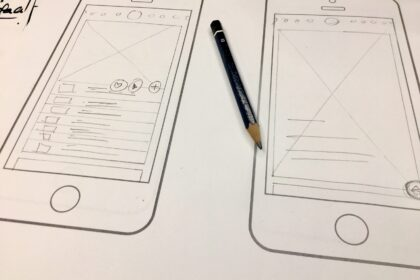# Symmetry vs Asymmetry: The Basic Design Principles

By Yumnatype on 1st February 2023

Symmetry and asymmetry are two different concepts in design and art. The concept of symmetry is based on the equal principle, in which an object or image is divided into two equal parts. Meanwhile, asymmetry is based on the unequal principle, in which an object or image is not divided into two equal parts.

This article will explore the differences and applications of symmetry and asymmetry in various fields.## Symmetry

A symmetrical design is a design form in which an object or image is divided into two exactly the same, equal parts. A symmetrical design can be applied in various fields, such as architecture, art, graphics, and others.

The example in art is a painting divided into two equal parts with the same shapes as in the Mona Lisa painting, or in graphic art for example a logo or poster divided into two equal parts.

A symmetrical design can also be applied in web designs or product designs. For example, headers and footers can be grouped by symmetry in website design, or a product design divided into two equal parts.

In general, a symmetrical design is considered a form of harmonious, aesthetic design as the two equal parts create a balanced, soothing visual perception.

There are several types of symmetry as follows.

1. Curved Symmetry. When an object is divided into equal halves, the two halves are exactly the same.
2. Linear Symmetry. When an object is divided equally, the two halves are exactly the same and stand perpendicular to the division line.
3. Radial Symmetry. When an object is divided into equal parts, each part is exactly the same and stands perpendicular to the center connecting line of the object to each part of the object.

## Asymmetry

Asymmetry is a term used to describe an object or shape that is not the same when divided into two equal parts.

In design, asymmetry is used to create unbalanced, asymmetrical compositions. Asymmetrical designs can add unique, expressive impressions, but may lack harmony. In graphic design, asymmetry can be used to create an asymmetrical poster or logo. In architecture, asymmetry is used to create asymmetrical, unbalanced composition buildings.

In general, asymmetry is considered a unique, dynamic, expressive form compared to the harmonious, aesthetic symmetry. However, asymmetry can also give an unbalanced, disharmonious impression if used improperly.

## Symmetrical and Asymmetrical Differences

Symmetrical and asymmetrical are two different concepts in design and art. The main differences are as follows:

1. Basic principle. Symmetry is based on the equal principle, in which an object or image is divided into two exactly equal parts. Meanwhile, asymmetry is based on the principle of unequality, in which an object or image is not divided into two exactly the same parts.
2. Visual impression. Symmetrical design is considered a harmonious, aesthetic form, as the two equal parts create a balanced and soothing visual perception. While an asymmetrical design is considered a unique, dynamic, expressive form, which may not give the impression of harmony. However, if you can compose it correctly, the visual will still look attractive.
3. Application. Symmetry can be applied in various fields, such as architecture, art, graphics, and others, while asymmetry is applied in art, architecture, graphics, product design, and other fields. Mostly they are used similarly, but it all depends on which design form suits you well.
4. Balance. Symmetrical can give a balanced, harmonious impression. Asymmetrical can give a dynamic, unique, unbalanced impression but that is what makes the design look more attractive.
5. Effect. Symmetry usually makes objects look more stable and formal, while asymmetry gives a more dynamic, expressive impression. However, this is not always true as both can be used in creative ways to achieve different effects.

## Recent Posts### The Importance of UI and UX### Things You Need to Know about a Storyboard